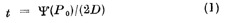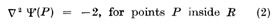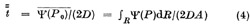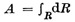# Torsion and other Mean First-passage Time Analogies

## Abstract

IN an attempt to cover the known mean first-passage time formulæ by Furth1 and others by a general theory of first-passage times of diffusing (Brownian) particles, some new formulæ and some general results were derived in a recent paper2. It was shown there that the average time taken by a particle starting from a point P 0 in a given region R to reach the boundary B of R is, in slightly changed notation,whereandHere D is the appropriate diffusion coefficient (it could refer to rotational diffusion) and 2 is the Laplacian operator appropriate to the space considered. Further, if the initial position of the particle is not specified but is equally likely anywhere within R,whereis the total extent of the region (area or volume, whichever is applicable).

## Access options

from\$8.99

All prices are NET prices.

## References

1. 1

Fürth, R., Ann. Phys., 53, 177 (1917).

2. 2

Klein, G., Proc. Roy. Soc., A, 211, 431 (1952).

3. 3

Prandtl, L., Phys. Z., 4, 758 (1903).

4. 4

Southwell, R. V., “Theory of Elasticity”, 2nd ed. (Oxford Univ. Press, 1953).

5. 5

Sokolnikoff, I. S., “Mathematical Theory of Elasticity”, 2nd ed. (McGraw-Hill, 1956).

## Rights and permissions

Reprints and Permissions